Electron. J. Diff. Equ., Vol. 2015 (2015), No. 278, pp. 1-9.

### Basicity in Lp of root functions for differential equations with involution Leonid V. Kritskov, Abdizhahan M. Sarsenbi

Abstract:
We consider the differential equation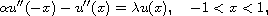with the nonlocal boundary conditions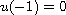,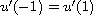where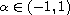. We prove that if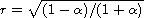is irrational then the system of its eigenfunctions is complete and minimal in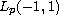for any, but does not constitute a basis. In the case of a rational value of r we specify the way of choosing the associated functions which provides the system of all root functions of the problem forms a basis in.

Submitted October 17, 2015. Published November 4, 2015.
Math Subject Classifications: 34K08, 34L10, 46B15.
Key Words: ODE with involution; nonlocal boundary-value problem; basicity of root functions.

Show me the PDF file (235 KB), TEX file, and other files for this article.Leonid V. Kritskov Lomonosov Moscow State University Faculty of Computational Mathematics and Cybernetics 119899 Moscow, Russia email: kritskov@cs.msu.ru Abdizhahan M. Sarsenbi Auezov South-Kazakhstan State University Department of Mathematical Methods and Modeling 160012 Shymkent Kazakhstan email: abzhahan@mail.ru